International
Tables for
Crystallography
Volume C
Mathematical, physical and chemical tables
Edited by E. Prince

International Tables for Crystallography (2006). Vol. C, ch. 5.2, pp. 491-492

Section 5.2.1. Introduction

W. Parrish,a A. J. C. Wilsonb and J. I. Langfordc

aIBM Almaden Research Center, San Jose, CA, USA,bSt John's College, Cambridge CB2 1TP, England, and cSchool of Physics & Astronomy, University of Birmingham, Birmingham B15 2TT, England

5.2.1. Introduction

| top | pdf |

5.2.1.1. The techniques available

| top | pdf |

X-ray powder methods for the accurate determination of lattice parameters can be divided broadly into four groups, depending on the type of dispersion, type of source, and the type of detector. They are:

 (1) angle-dispersive: diffractometer methods, conventional tube source (Section 2.3.2); (2) angle-dispersive: diffractometer methods, synchrotron sources (Subsections 2.3.3.1, 2.3.3.2); (3) energy-dispersive: diffractometer methods (Subsection 2.3.3.3, Chapter 2.5); (4) angle-dispersive: camera methods (Section 2.3.4).

The geometry, advantages, and some practical details of the methods are given in the sections whose numbers are given in parentheses. The techniques will be discussed in the above order in Sections 5.2.4–5.2.8. More details of systematic errors in diffractometry are given in Wilson (1963, 1965c, 1974). Some general points on checking precision and accuracy were made in Chapter 5.1. Many of them are treated in greater detail in Section 2.3.5, and are recapitulated in Section 5.2.13.

The technique of choice will depend on the accuracy required and on the nature and quantity of the material available. At present, the technique most frequently used for the purposes of this chapter is angle-dispersive diffractometry with a conventional tube source (1). Angle-dispersive diffractometry with synchrotron radiation (2) is capable of greater precision and accuracy, but access to the synchrotron sources is cumbersome and may involve long waiting periods. Energy-dispersive methods (3) would ordinarily be adopted only if the required environmental conditions (high or low temperatures, high pressures,) can be achieved most readily by means of a fixed-angle diffractometer. Camera methods (4) are adaptable to small quantities of material, but microdiffractometers (Subsection 2.3.1.5) can be used with similar or even smaller quantities.

5.2.1.2. Errors and aberrations: general discussion

| top | pdf |

The relation between the lattice spacing d, the angle of incidence (Bragg angle) θ, and the wavelength λ is Bragg's law: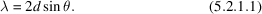The lattice spacing d is related to the lattice parameters a, b, c, α, β, γ and the indices of reflection h, k, l. In the simple case of cubic crystals, the relation is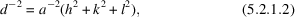where a is the single lattice parameter. The general relation is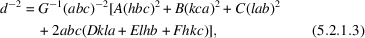where a, b, c are the edges of the unit cell, and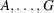are the functions of the angles of the unit cell given in Table 5.2.1.1.

 Table 5.2.1.1| top | pdf | Functions of the cell angles in equation (5.2.1.3)for the possible unit cells
FunctionCell
Cubic
tetragonal
orthorhombic
HexagonalMonoclinic (c unique)RhombohedralTriclinic
A 1 1 1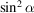B 1 1 1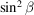C 1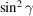D 0 0 0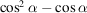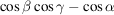E 0 0 0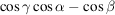F 0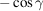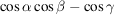G 1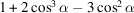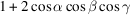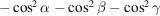Differentiation of (5.2.1.1)shows that the errors in the measurement of d are related to the errors in the measurement of λ and θ by the equation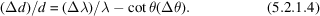Wavelength and related problems are discussed in Section 5.2.2and geometrical and other aberration problems in Section 5.2.3.

5.2.1.3. Errors of the Bragg angle

| top | pdf |

The error in the Bragg angle,, will ordinarily consist of both random and systematic components. The random components (as the name implies) have an expected value zero, but the systematic errors will affect all measurements consistently to a greater or lesser extent. The systematic errors may be, and usually are, functions of θ and/or λ. Such errors would ordinarily reveal themselves in checks of internal consistency: the values of the apparent lattice parameter, plotted as a function of θ, would show a systematic drift, not a random scatter. The success or otherwise of attempts to eliminate or account for them would be subject to statistical tests (Section 5.2.9and Chapter 8.5). There is an exception to the ordinarily'; if the variation ofwith θ happens to be of the form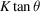, where K does not depend on θ either explicitly or through λ, the resultant fractional error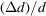is a constant, and would not be revealed either by systematic drift of the apparent lattice parameter with θ or by statistical tests.

5.2.1.4. Bragg angle: operational definitions

| top | pdf |

The Bragg angles are determined from the observations by a series of operations that are often quite complex.

For film cameras of diameter 57.3 or 114.6 mm, a simple measurement with a millimetre scale gives θ in degrees (1 mm = 1 or 0.5°). This determination is crude, and ordinarily the lines on the film would be measured with a low-power travelling microscope or a densitometer. The effective camera diameter is found from measurements of fiducial marks imprinted on the film, or by use of the Straumanis film mounting. References to detailed descriptions are given in Section 2.3.4.

For Bragg–Brentano (Parrish) and Seemann–Bohlin diffractometers, rate-meter measurements with strip-chart recordings have time-constant errors, and precision measurements require step-scanning (Subsection 2.3.3.5). The data may be analysed to give one or more of the following measures of position:

 (a) The centroid of the reflection (Subsection 2.3.3.3). (b) The peak of the reflection (Subsection 2.3.3.3). The extrapolated mid-point of chords is a kind of modified peak determination, but the best method of locating peaks so far in operation is that called peak search' (Subsection2.3.3.7). (c) Profile fitting (Subsection 2.3.3.8). In principle, profile fitting could give the Bragg angle corresponding to any desired feature of the diffraction maximum (centroid peak, median,), but it has been used in practice mainly for locating the Bragg angle corresponding to the peak.

As usual, it is necessary to distinguish between the precision (reproducibility) of a measurement and its accuracy (extent to which it is affected by systematic errors). In principle, it does not matter if the Bragg angle obtained by any of the above operations is affected by systematic errors, as these can be calculated and allowed for, as described in the following paragraphs. The most precise methods are the peak-search and individual profile-fitting computer procedures. They are routinely capable of a precision of about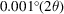for reasonably sharp reflections, and are free from the subjective effects that may influence, for example, film measurements or the graphical extrapolation of the mid-points of chords. As well as a measure of the peak position, the peak-search procedure gives a measure of the peak intensity, and the profile-fitting procedure gives a measure of the peak intensity and (if desired) a measure of the integrated intensity.

References

Wilson, A. J. C. (1963). Mathematical theory of X-ray powder diffractometry. Eindhoven: Centrex.
Wilson, A. J. C. (1965c). Röntgenstrahlpulverdiffractometrie. Mathematische Theorie. Eindhoven: Centrex.
Wilson, A. J. C. (1974). Powder diffractometry. X-ray diffraction, by L. V. Azaroff, R. Kaplow, N. Kato, R. Weiss, A. J. C. Wilson & R. A. Young, Chap. 6. New York: McGraw-Hill.